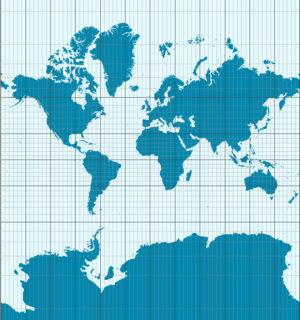# Theorema egregium facts for kids

Kids Encyclopedia FactsA consequence of the Theorema Egregium is that the Earth cannot be displayed on a map without distortion. The Mercator projection, shown here, preserves angles but changes the area. As an example, Antarctica is shown much bigger than it really is.

Gauss's Theorema Egregium (Latin for "Remarkable Theorem") is a major result of differential geometry proved by Carl Friedrich Gauss. The theorem is about the curvature of surfaces. The theorem states that curvature can be determined by measuring angles, distances and their rates on a surface alone. There is no need to talk about the particular manner in which the surface is embedded in the surrounding three-dimensional Euclidean space. In other words, the Gaussian curvature of a surface does not change if one bends the surface without stretching it.

Gauss presented the theorem in this manner (translated from Latin):

For this reason the formula of the preceding article leads itself to the remarkable Theorem. If a curved surface is developed on any other surface whatever, the measure of curvature in each point remains unchanged.

The theorem is "remarkable" because the starting definition of Gaussian curvature makes direct use of the position of the surface in space. So it is quite surprising that the result does not depend on its embedding in spite of all bending and twisting deformations undergone.

## Images for kidsTheorema egregium Facts for Kids. Kiddle Encyclopedia.